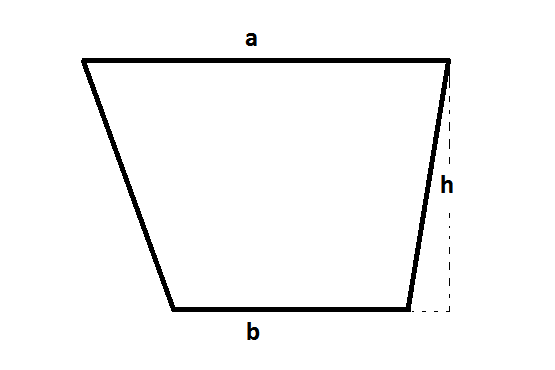# Centroid of a Trapezoid Formula

A trapezoid is a quadrilateral with two sides parallel. The centroid of a trapezoid lies between the two bases. For any trapezoid with parallel sides a and b, use below given formula.Find below formula for the centroid of trapezoid located a distance of x,

$\LARGE x = \frac{b+2a}{3(a+b)}h$

Where,

h = Height of trapezoid

a, b = Length of parallel sides

### Solved Examples

Question 1: Find the centroid of trapezoid with the given dimensions; a = 12′ ; b = 5′ ; h = 5′

Solution: Given,

a = 12′; b = 5′; h = 5′

Using centroid of trapezoid formula,

x =

$$\begin{array}{l}\frac{b + 2a}{3(a + b)}\end{array}$$
× h

x =

$$\begin{array}{l}\frac{5 + 2 \times 12}{3(12 + 5)}\end{array}$$
× 5

x = 2.84

Hence, the centroid of the trapezoid is at a distance of 2.84′.

Example 2:

If the parallel sides of trapezoid measures 8 cm, 10 cm and the height 9 cm, then find its centroid.

Solution:

Let a and b be the parallel sides of a trapezoid.

According to the given,

a = 8 cm, b = 10 cm and h = 9 cm

Using the centroid of trapezoid formula,

$$\begin{array}{l}x=\frac{b+2a}{3(a+b)}\times h\\=\frac{10+2(8)}{3(8+10)}\times 9\\=\frac{26}{54}\times 9\\=\frac{13}{3}\\=4.33\end{array}$$